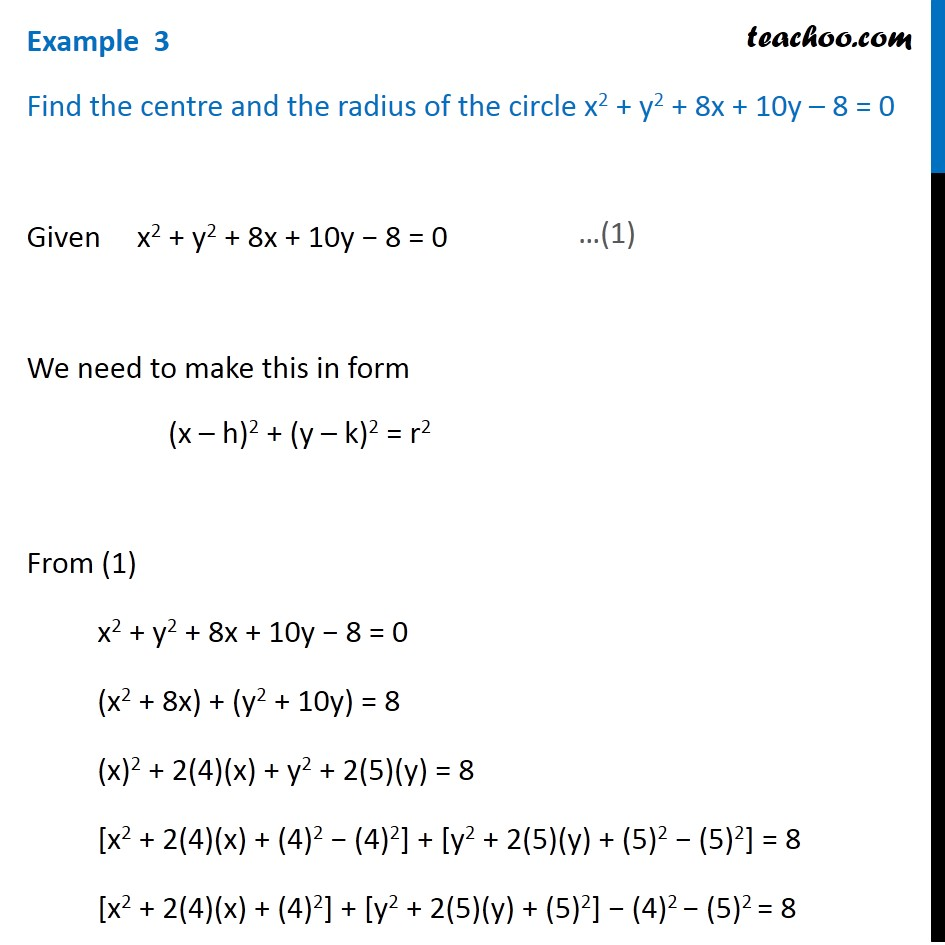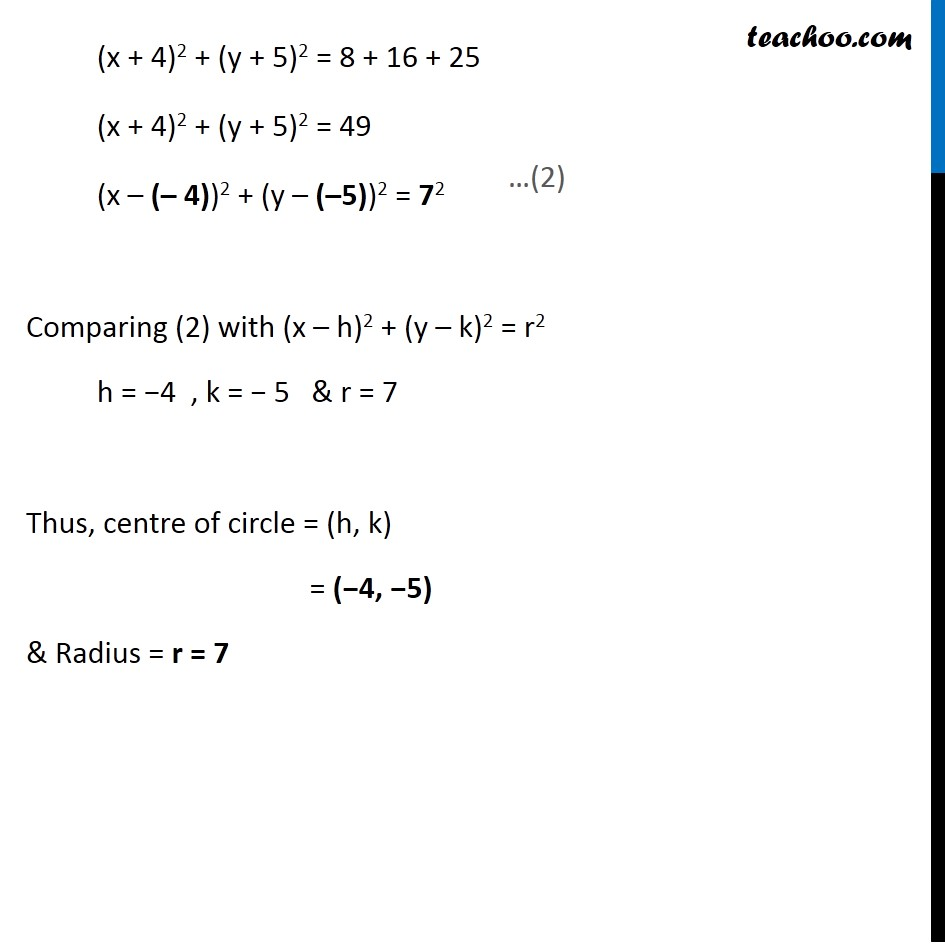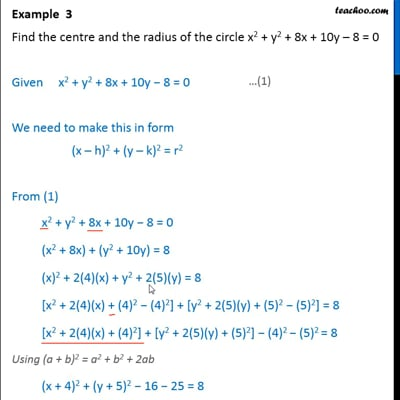Circle

Chapter 11 Class 11 Conic Sections
Concept wiseThis video is only available for Teachoo black users

Introducing your new favourite teacher - Teachoo Black, at only ₹83 per month

### Transcript

Example 3 Find the centre and the radius of the circle x2 + y2 + 8x + 10y – 8 = 0 Given x2 + y2 + 8x + 10y − 8 = 0 We need to make this in form (x – h)2 + (y – k)2 = r2 From (1) x2 + y2 + 8x + 10y − 8 = 0 (x2 + 8x) + (y2 + 10y) = 8 (x)2 + 2(4)(x) + y2 + 2(5)(y) = 8 [x2 + 2(4)(x) + (4)2 − (4)2] + [y2 + 2(5)(y) + (5)2 − (5)2] = 8 [x2 + 2(4)(x) + (4)2] + [y2 + 2(5)(y) + (5)2] − (4)2 − (5)2 = 8 (x + 4)2 + (y + 5)2 = 8 + 16 + 25 (x + 4)2 + (y + 5)2 = 49 (x – (– 4))2 + (y – (–5))2 = 72 Comparing (2) with (x – h)2 + (y – k)2 = r2 h = −4 , k = − 5 & r = 7 Thus, centre of circle = (h, k) = (−4, −5) & Radius = r = 7